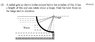# Fluid Mechanics question

• AshNZ
In summary, the Radial gate has a radius of 6 meters, a length of 8 meters, and can rotate about a hinge. The force on the hinge is the sum of the vertical and horizontal forces. The resultant force is directed at zero degrees.

#### AshNZ

Hey guys,

I'm new here. I've been following PF on and off to get some hints in past but this is the first time I'm posting. I'm doing civil engineering second year and the following is an assignment question (there are total of four questions and I'm stuck on all four :( ). I'm not looking for direct answers but any hints would be great.

Q: Radial gate has a radius of 6m. It has length of 8m and can rotate about a hinge. What's total force on the hinge and its direction?

The book I usually refer to uses the following procedure:
First they find Fh using rho*g*h
Then they find Fv using rho*g*V where V is the volume of water above the surface of the surface of the gate. And then they go on to some other formulas but I'm stuck at this point because in this particular question there is no water above the surface of the gate, which makes Fv = 0 (which inevitably leads to resultant force's direction of zero degrees in later stages)

I could be knocking on the door of the answer or I could be in the other city. Any help will be appreciated.

Last edited:
Can you show a picture?

There isn't a lot in the picture but here we go..

#### Attachments

•FluidMechQuestion.jpg
27.3 KB · Views: 391
I've cracked this question. There was a similar question in past years exam papers and luckily I had the solution for that paper.

I am assuming for anyone in the future searching for this thread...

You break the load into two components, one horizontal, and one vertical. You then integrate the pressure $$\rho g h$$ along with the equation for a circle to get the total load in each direction?

Yes, you break it down to horz and vertical. Then find horizontal by rho*g*h*A(projected area). Then you find vertical using )(pi*r^2L)/4)*rho*g. Then use trig to find resultant and the angle.